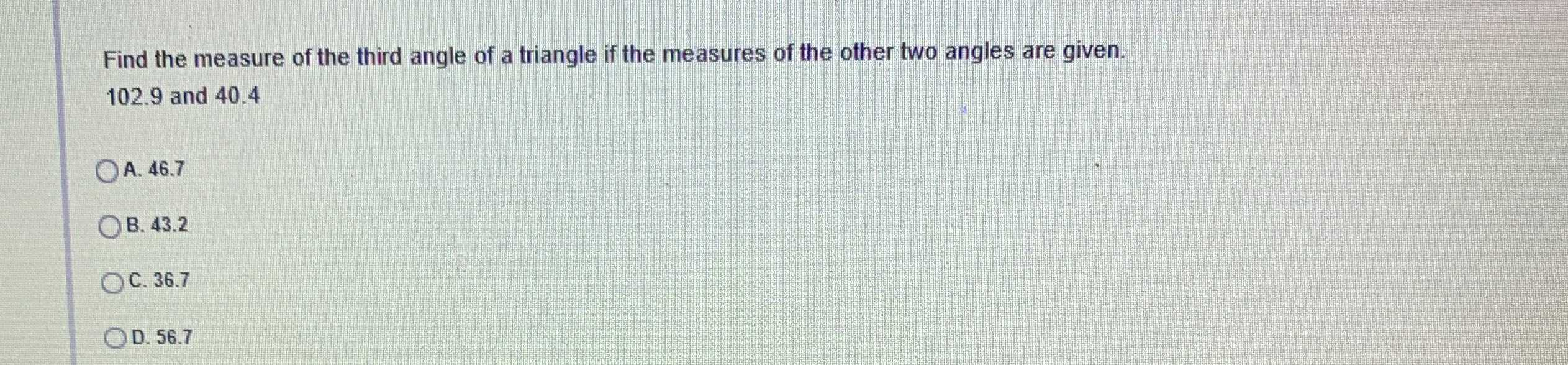### Still have math questions?

Trigonometry
QuestionFind the measure of the third angle of a triangle if the measures of the other two angles are given.

$$102.9$$ and $$40.4$$

A. $$46.7$$

B. $$43.2$$

C. $$36.7$$

D. $$56.7$$